﻿ Condition Builder
 Operations > Strategy Wizard > Condition Builder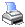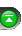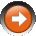The Condition Builder is a very powerful feature that allows you to define complex conditions for your automated trading systems without having to know how to program.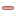Understanding the Condition Builder
 Condition Builder Most if not all automated trading system code wizards are limited in scope in that they provide canned pre-defined expressions and only allow you to change a few parameters on those expressions. The NinjaTrader Condition Builder is advanced in that you can develop powerful expressions without limitations. Due to its power and flexibility, it is extremely important that you read through and understand its capabilities.   The Condition Builder is also a very powerful aid for those of you learning NinjaScript or learning how to program. You can build your conditions within the Condition Builder and instantly see NinjaScript code generated by having the NinjaScript Editor open ( by pressing the View Code... button in the wizard screen). You can also use the Condition Builder from within the NinjaScript Editor directly. By doing so, NinjaScript code is automatically generated and inserted into your code.   The Condition Builder can be accessed via the Conditions and Actions wizard screen or the right mouse button click menu of the NinjaScript Editor.   Basic Operation The general concept of the Condition Builder to generate a Boolean expression also known as comparison expressions or conditional expressions. What does that mean? It is simply an expression that results in a value of either TRUE or FALSE. For example, the expression   2 < 7 (2 is less than 7)   is a Boolean expression because the result is TRUE. All expressions that contain relational operators are Boolean. Boolean expressions or "Conditions" as they are known in NinjaTrader is used to determine when to take a specified action such as submitting an order or drawing on the chart.   Looking at the image below, you can instantly see that the Condition Builder is set up like a Boolean expression. Select an item from the left window, select the relational operator (2) and compare it to a selected item in the right window.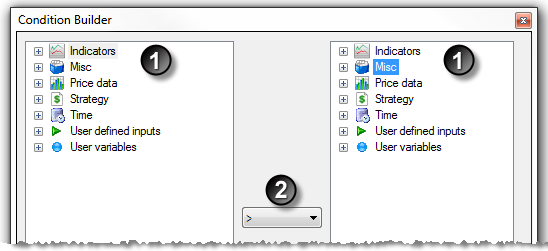1. Available items such as indicators, price data, etc. to use for the comparison 2. List of relational operatorsHow to make price data comparisons

Price Data Comparisons

You can compare a bar's price data such as checking for a higher close. The following is an an example and represents one of many possible combinations.

 1 Expand the Price data category and select the Close.
 2 Select the > (greater than) relational operator
 3 Expand the Price data category and select the Close.
 4 Set the Bars ago parameter to a value of "1"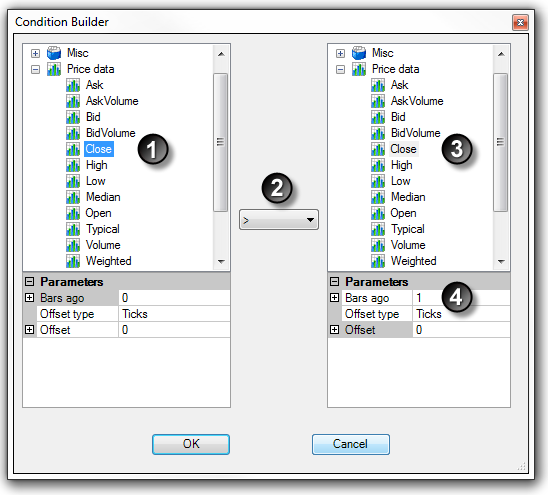Once the OK button is pressed, a condition is created that would translate to the following:

"Current closing price is greater than the closing price of 1 bar ago"How to offset an item value

Offsetting an Item Value

You can offset the value of most items available in the Condition Builder. An offset is a value that is added or subtracted from the actual item's value. When an item is selected such as an indicator or price data, the Offset type and Offset parameters become visible in the window directly below the item selected. This is shown as numbers 5 and 6 in the image below.

Offset type can be set to:

 Percent Adds or subtracts a percentage value of the item's value. A value of 1 is equal to 100% where a value of 0.1 is equal to 10%. Price Adds or subtracts an absolute value Ticks Adds or subtracts the number of ticks (0.01 for stocks and the tick size for futures or currencies) from the item's value

Once the Offset type is selected, you must set the value Offset. In addition to the example below, you can see the "Checking for Volume Expansion" section below for another example that uses the Percent Offset type.

The following is an example and represents one of many possible combinations:

1. Expand the Price data category and select the Close

2. Select the > (greater than) relational operator

3. Expand the Price data category and select the High

4. Set the Bars ago parameter to a value of "1"

5. Set the Offset type parameter to Ticks

6. Set the Offset parameter to a value of "1"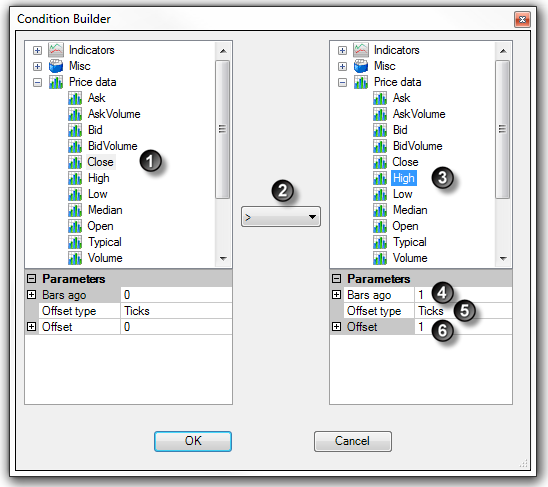Once the OK button is pressed, a condition is created that would translate to the following:

"Current closing price is greater than the high price of 1 bar ago + 1 tick"How to make indicator to value comparisons

Indicator to Value Comparisons

You can compare an indicator's value to a numeric value. This can come in handy if you wanted to check if ADX is over a value of 30 (trending) or if Stochastics is under a value of 20 (oversold) or any other conditions you can think of.

The following is an an example and represents one of many possible combinations:

 1 Expand the Indicators category and select the ADX indicator
 2 Set the item parameters
 3 Select the > (greater than) relational operator
 4 Expand the Misc category and select Numeric value
 5 Enter the numeric value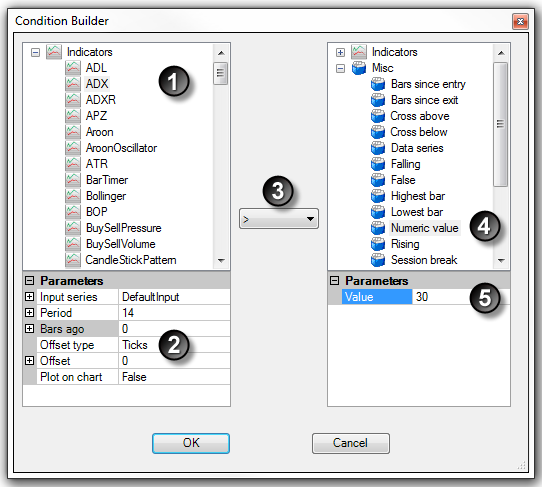Once the OK button is pressed, a condition is created that would translate to the following:

"Current value of a 14 period ADX is greater than 30"How to compare plot values of multi-plot indicators

Comparing Plot Values of Multi-Plot Indicators

You can compare plots in the same indicator or select any individual plot within an indicator to create a condition.

The following is an example and represents one of many possible combinations.

 1 Expand the Indicators category and select the Stochastics indicator
 2 Set the indicator input parameters and select the K plot (blue arrow)
 3 Select the > (greater than) relational operator
 4 Expand the Indicators category and select the Stochastics indicator
 5 Set the indicator input parameters and select the D plot (blue arrow)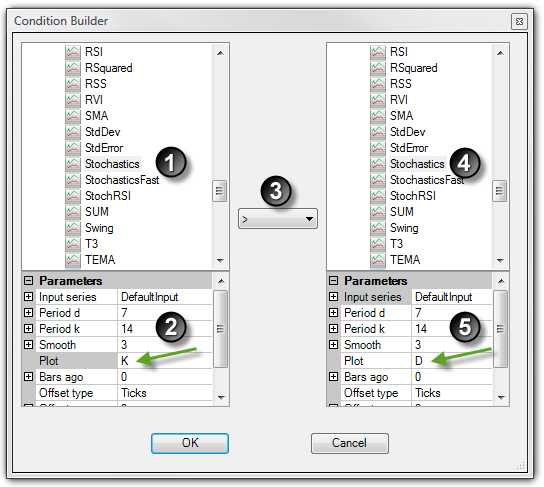Once the OK button is pressed, a condition is created that would translate to the following:

"Current K plot value of a Stochastics indicator is greater than the current D plot value of the same Stochastics indicator"How to use user defined inputs & variables

User Defined Inputs & Variables

User defined inputs are simply variables that can be used in place of absolute values. They increase the flexibility of your strategy since you can substitute a variable for the period parameter of a simple moving average instead of provide an absolute value.

SMA(9) is how you express a 9 period simple moving average in NinjaScript. If you run a strategy, you would always be using a 9 period simple moving average. At run time, you might want to change this value to 10. User defined inputs accomplish this. If you created an input named "MyInput", you could express the simple moving average as SMA(MyInput). At run time, you can then configure your strategy by setting the value of "MyInput" to whatever value you like. In addition, user defined inputs are required when optimizing a strategy.

User variables (not to be confused with inputs) behave in the same manner with the exception that they can not be configured when starting a strategy but can only be set programmatically during run time.

 • User defined inputs are created from the wizard screen
 • User variables can be set in the strategy logic through the Condition Builder (see the sections above)

The following is an an example and represents one of many possible combinations. The example demonstrates the user of a user defined input however the sample approach applies to user variables.

 1 Expand the Price data category and select the Close.
 2 Select the > (greater than) relational operator
 3 Expand the Indicators category and select the SMA indicator
 4 Set the Period parameter to a user defined input by pressing the "..." button to open the Value window
 5 Expand the User defined inputs category and select the value MAPeriod and press the OK button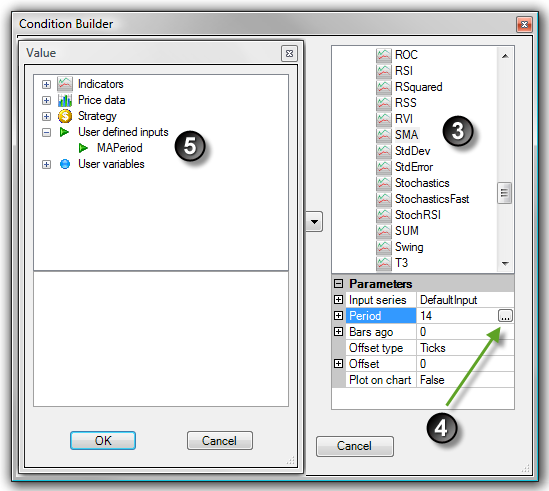6 The Condition Builder will look as per the image below with the user defined input "MAPeriod" assigned to the parameter Period. When you apply this strategy to a chart, you will be able to set the value for the user defined input which will then be used to drive the SMA indicator.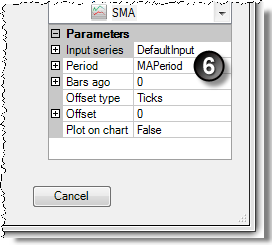Once the OK button is pressed, a condition is created that would translate to the following:

"Current closing price is greater than the user defined Period simple moving average"How to create a cross over condition
 Cross Over Conditions You can check for either a CrossAbove or CrossBelow condition with a user defined look back period. The look back period sets the number of bars to look back to check for the cross over condition.   The following is an an example and represents one of many possible combinations.   1. Expand the Indicators category and select the EMA indicator 2. Set the Period parameter to a value ("9" is used in this example) 3. Select CrossAbove 4. Set the Look back period 5. Expand the Indicators category and select the EMA indicator 6. Set the Period parameter to a value ("20" is used in this example)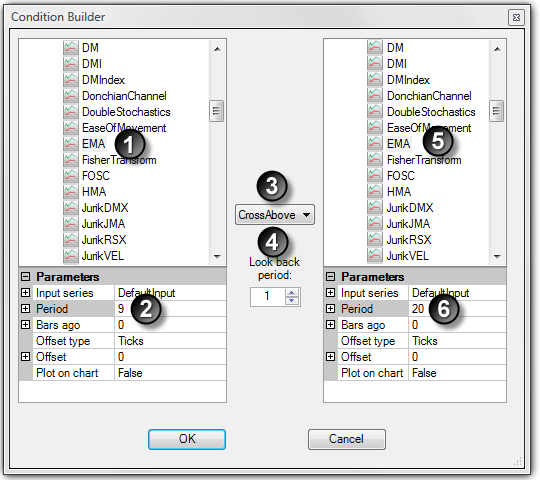Once the OK button is pressed, a condition is created that would translate to the following:   "9 period exponential moving average crosses above the 20 period exponential moving average in the last bar"How to use indicator inputs in other indicators

Indicator on Indicator

You can use indicators as input for other indicators... actually, you can nest indicators within indicators infinitely if you really wanted to!

The following example is an example of applying a simple moving average (SMA) to a 14 period ADX indicator and is one of many possible combinations.

 1 Expand the Indicator category and select SMA indicator
 2 Set Input series to the ADX indicator by pressing the "..." button to open the Value window
 3 Select the ADX indicator and set any properties in the Parameters window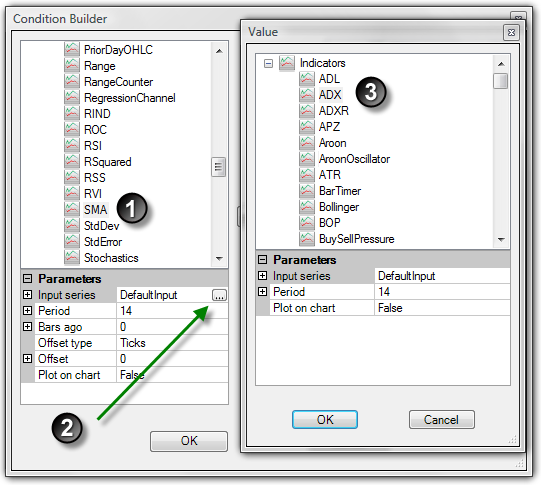4 Press the OK button5 Once you have pressed the OK button, you will notice on the left lower window, the "Input series" parameters has now been set to the ADX(14) which is the 14 period ADX indicator.

Once the OK button is pressed, a condition is created that would translate to the following:

"Current value of a 14 period simple moving average of a 14 period ADX is greater than 30"How to check for volume expansion

Checking for Volume Expansion

You can compare if the current bar's volume is greater than the prior bar's volume plus an offset amount.

The following is an an example and represents one of many possible combinations.

 1 Expand the Indicators category and select the VOL indicator
 2 Select the >= (greater than or equal to) relational operator
 3 Expand the Indicators category and select the VOL indicator
 4 Set the Bars ago parameter to a value of "1"
 5 Set Offset type parameter to Percent
 6 Set the Offset parameter to a value of "3"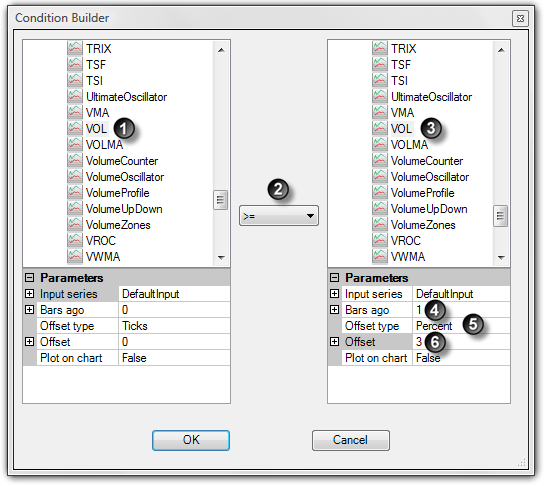Once the OK button is pressed, a condition is created that would translate to the following:

"Current value of Volume is greater than or equal to the value of Volume of 1 bar ago + 300%"How to create market position comparisons

Creating Market Position Comparisons

You can compare a strategy state information such as but not limited to market position or position size.

The following is an an example and represents one of many possible combinations.

 1 Expand the Strategy category and select Current market position.
 2 Select the == (equals to) relational operator
 3 Expand the Strategy category and select Flat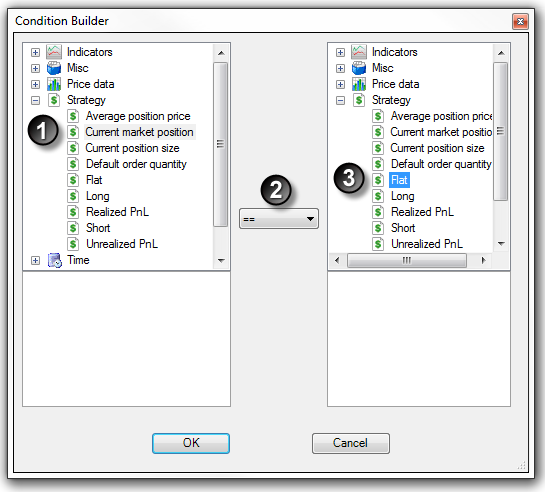Once the OK button is pressed, a condition is created that would translate to the following:

"Current market position equals flat"How to create time comparisons
 Creating Time Comparisons You can compare a bar's time data to a user defined time or date value.   The following is an an example and represents one of many possible combinations.   Note: Time series represents a collection of bar Date/Time values of a bar series   1. Expand the Time category and select Time series 2. Select the >= (greater than or equal to) relational operator 3. Expand the Time category and select Time value 4. Set the Value parameter to a user defined value of "10:00 AM"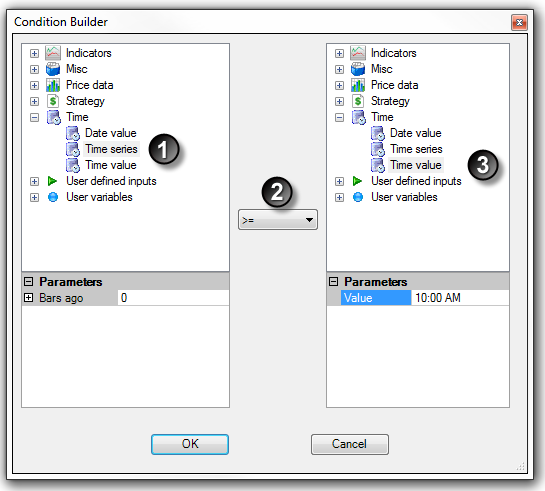Once the OK button is pressed, a condition is created that would translate to the following:   "Current bar's time is greater or equal to 10:00 AM"